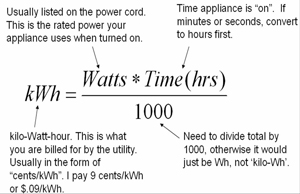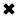# What is a Watthour?#### A Watthour is equivalent to one watt of power, dissipated over a period of time.

The watt-hour (Wh) is a unit of energy equivalent to a single watt (1 Watt) of electricity expended for duration of one hour (1 hour). While the watthour is not a standard unit in any formal system, it is commonly used in electrical applications.

This is because there is an important difference between energy and power. You've probably heard the two terms used interchangeably, as if they mean the same thing. But they don't mean the same thing. Energy is power dissipated over a length of time. Power is the rate at which energy is expended.

Physicists measure energy in joules. One joule is the equivalent of one watt of power, dissipated for one second of time. In electricity, you'll more often encounter the watt hour or the kilowatt hour. As their names imply, a watt hour, abbreviated Wh, is the equivalent of 1 W dissipated for an hour (1 h), and 1 kilowatt hour (kWh) is the equivalent of 1 kW of power dissipated for 1 hour.

An energy of 1 Wh can be dissipated in an infinite number of different ways. A 60-watt bulb will bum 60 Wh in an hour, or 1 Wh per minute. A 100-W bulb would bum 1 Wh in lhoo hour, or 36 seconds. A 6-watt Christmas tree bulb would require 10 min­utes (Ve hour) to bum 1 Wh. And the rate of power dissipation need not be constant; it could be constantly changing.Fig. 2-6. Two hypothetical devices that consume 1 Wh of energy.

Figure 2-6 illustrates two hypothetical devices that bum up 1 Wh of energy. Device A uses its power at a constant rate of 60 watts, so it consumes 1 Wh in a minute. The power consumption rate of device B varies, starting at zero and ending up at quite a lot more than 60 W. How do you know that this second device really bums up 1 Wh of en­ergy? You determine the area under the graph. This example has been chosen because figuring out this area is rather easy. Remember that the area of a triangle is equal to half the product of the base length and the height. This second device is on for 72 seconds, or 1.2 minute; this is 1.2/60 = 0.02 hour. Then the area under the "curve" is \l•i x 100 x 0.02 = 1 Wh.

When calculating energy values, you must always remember the units you're using. In this case the unit is the watt hour, so you must multiply watts by hours. If you multi­ply watts by minutes, or watts by seconds, you'll get the wrong kind of units in your answer. That means a wrong answer!

Sometimes, the curves in graphs like these are complicated. In fact, they usually are. Consider the graph of power consumption in your home, versus time, for a whole day. It might look something like the curve in Fig. 2-7. Finding the area under this curve is no easy task, if you have only this graph to go by. But there is another way to deter­mine the total energy burned by your household in a day, or in a week, or most often, in a month. That is by means of the electric meter. It measures electrical energy in kilowatt hours. Every month, without fail, the power company sends its representative to read that meter. This person takes down the number of kilowatt hours displayed, subtracts the number from the previous month, and a few days later you get a bill. This meter au­tomatically keeps track of total consumed energy, without anybody having to do so­phisticated integral calculus to find the areas under irregular curves such as the graph of Fig. 2-7.Fig. 2-7. Graph showing the amount of power consumed by a hypothetical household, as a function of the time of day.

##### Basic Electricity Handbook, Vol. 1• FULLY ILLUSTRATED
• GREAT FOR SCHOOL PROJECTS
• GREAT PRICE: \$5.99
• ...

This 100+ page e-book is a great guide for those who have a basic interest in the field of electricity. This well-illustrated e-book, coupled with some basic knowledge of electricity, will give you a broad theoretical background in this fundamental subject.

CONTENTS
• FUNDAMENTALS OF ELECTRICITY
• HOW DOES ELECTRICITY WORK?
• HOW IS ELECTRICITY GENERATED?
• WHERE DOES ELECTRICITY COME FROM?
• BASIC HOME WIRING
• ELECTRICITY FOR STUDENTS
• ENERGY SAVING TIPS

##### TRAINING EF COURSES

Content Community Connection
Top